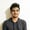# Trigonometry, Random Numbers and More With Built-in PHP Math FunctionsBasic maths is used a lot during programming. We need to frequently compare, add, multiply, subtract and divide different values when writing code.

Sometimes, the maths required in a program can be more involved. You might need to work with logarithmic, trigonometric or exponential functions. In this tutorial, I'll discuss how to use each of these functions in PHP, with examples.

This tutorial will introduce you to the built-in math functions in PHP for doing trigonometry, exponentiation, and logarithm calculations. We'll also look at rounding and generating random numbers.

## Trigonometric Functions in PHP

### Minimum and Maximum

PHP also has two functions called min($values) and max($values) to help you determine the lowest and highest values in a set or array of numbers. These functions can accept different kinds of parameters like two arrays and a string. You should take a look at the documentation to see how they would be compared.

### Integer Division

You can also perform integer division in PHP using the intdiv($dividend,$divisor) function. In this case, only the integral part of the quotient is returned after a division. Similarly, you can also get the remainder or modulo after the division of two arguments using the fmod($dividend,$divisor) function. The returned value will always be less than the $divisor in magnitude. There are some other useful functions like is_nan($value), is_finite($value) and is_infinite($val) which can be used to determine if the value is a number and, if it is a number, whether it is finite or infinite. Remember that PHP considers any value that is too big to fit in a float to be infinite. So is_finite() will return true for 100 factorial but false for 1000 factorial.

## Generating Random Numbers in PHP

Random numbers prove to be quite useful in a number of situations. You can use them to generate "random" data for your application or to spawn enemy instances in games, etc. It is very important to remember that none of the functions we discuss in this section generate cryptographically secure random numbers. These functions are only meant to be used in situations where security is not an issue, like showing random greeting texts to repeat visitors or for generating random statistical data.

The functions rand($min,$max) and mt_rand($min,$max) can generate positive random integers between given values including the $min and $max value. When the functions are called without any parameters, they generate random numbers between 0 and getrandmax(). You can echo the value of getrandmax() to see the maximum possible random number that these functions can generate on your platform.

The function mt_rand() is 4 times faster than rand() and returns false if $max is less than $min. Starting from PHP 7.1.0, rand() has actually been made an alias of mt_rand(). The only difference is that rand() still doesn't give an error if $max is less than $min to maintain backward compatibility.

Here is a loop to generate random values between 0 and 100 a million times. As you can see, the values 0, 50 and 100 are generated approximately 10,000 times with slight fluctuations.

Both these functions also have their own seeder functions called srand() and mt_srand() to provide a seed for the random number generators. You should just keep in mind that you only call srand() and mt_srand() once in your program. Calling them before every call to rand() and mt_rand() will give you the same "random" numbers every time.

## Final Thoughts

PHP comes with a lot of built-in functions that should meet all your day-to-day computation needs. You can use these functions to do slightly more complicated calculations like GCD, LCM and factorials yourself.

There are just a couple of things you should remember when using these functions. For example, the value returned by functions like floor() and ceil() is an integer, but it is still a float. Similarly, all trigonometric functions expect their angles to be given in radians. You will get incorrect results if you pass them an angle value that you wanted to be treated as a degree measure. So make sure you check the return value and expected arguments of all these functions in the documentation.